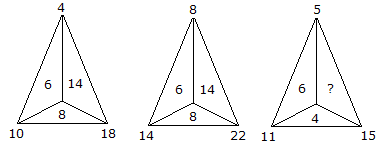# Verbal Reasoning - Character Puzzles - Discussion

### Discussion :: Character Puzzles - Character Puzzles 1 (Q.No.53)

53.

Which one will replace the question mark ?[A]. 8 [B]. 14 [C]. 10 [D]. 6

Explanation:

For first triangle,

10 - 4 = 6

18 - 10 = 8

18 - 4 = 14

For second triangle,

14 - 8 = 6

22 - 14 = 8

22 - 8 = 14

For third triangle,

11 - 5 = 6

15 - 11 = 4

15 - 5 = 10.

 Rahul said: (Jul 31, 2010) 8+6 = 14 8+6 = 14 4+6 = ? 4+6 = 10

 Mukund Saroj said: (Feb 3, 2019) 1) 4+6=10 4+14=18. 2) 8+6=14 8+14=22. 3) 5+6=11 5+?=15. So, 5+10 = 15.

 Ratikanta Nayak said: (Aug 4, 2021) Good, Thanks @Mukund Saroj.